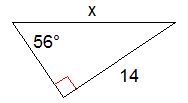# X006 Trigonometry - Find A Missing Side

10 Questions | Total Attempts: 70Settings• 1.
Find the length of the missing side, marked 'x', correct to one decimal place.
• 2.
Find the length of the missing side, marked 'x', correct to one decimal place.
• 3.
Find the length of the missing side, marked 'x', correct to one decimal place.
• 4.
Find the length of the missing side, marked 'x', correct to one decimal place.
• 5.
Find the length of the missing side, marked 'x', correct to one decimal place.
• 6.
Find the length of the missing side, marked 'x', correct to one decimal place.
• 7.
Find the length of the missing side, marked 'x', correct to one decimal place.
• 8.
Find the length of the missing side, marked 'x', correct to one decimal place.
• 9.
Find the length of the missing side, marked 'x', correct to one decimal place.
• 10.
Find the length of the missing side, marked 'x', correct to one decimal place.
• 11.
Find the length of the missing side, marked 'x', correct to one decimal place.
• 12.
Find the length of the missing side, marked 'x', correct to one decimal place.
• 13.
Find the length of the missing side, marked 'x', correct to one decimal place.
• 14.
Find the length of the missing side, marked 'x', correct to one decimal place.
• 15.
Find the length of the missing side, marked 'x', correct to one decimal place.
• 16.
Find the length of the missing side, marked 'x', correct to one decimal place.
• 17.
Find the length of the missing side, marked 'x', correct to one decimal place.
• 18.
Find the length of the missing side, marked 'x', correct to one decimal place.
• 19.
Find the length of the missing side, marked 'x', correct to one decimal place.
• 20.
Find the length of the missing side, marked 'x', correct to one decimal place.
• 21.
Find the length of the missing side, marked 'x', correct to one decimal place.
• 22.
Find the length of the missing side, marked 'x', correct to one decimal place.
• 23.
Find the length of the missing side, marked 'x', correct to one decimal place.
• 24.
Find the length of the missing side, marked 'x', correct to one decimal place.
• 25.
Find the length of the missing side, marked 'x', correct to one decimal place.
Related TopicsBack to top